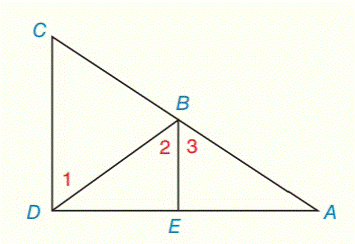Chapter 2.CR, Problem 35CRElementary Geometry For College St...

7th Edition
Alexander + 2 others
ISBN: 9781337614085

Solutions

Chapter
SectionElementary Geometry For College St...

7th Edition
Alexander + 2 others
ISBN: 9781337614085
Textbook Problem

Given: B E ¯ ⊥ D A ¯ C D ¯ ⊥ D A ¯ Prove: ∠ 1 ≅ ∠ 2To determine

To prove:

1 is congruent to 2.

Explanation

Given:

The given statement is,

BE¯DA¯ and CD¯DA¯

Figure (1)

Properties used:

(1) If two parallel lines are cut by a transversal, then the pairs of the alternate interior angles are congruent.

(2) If two lines are perpendicular to a same line, then the lines are parallel to each other.

Approach:

The given statement is,

BE¯DA¯ and CD¯DA¯

BE¯ and CD¯ both are perpendicular to the same line DA.

So,

BE¯CD¯

Still sussing out bartleby?

Check out a sample textbook solution.

See a sample solution

The Solution to Your Study Problems

Bartleby provides explanations to thousands of textbook problems written by our experts, many with advanced degrees!

Get Started

Find more solutions based on key concepts